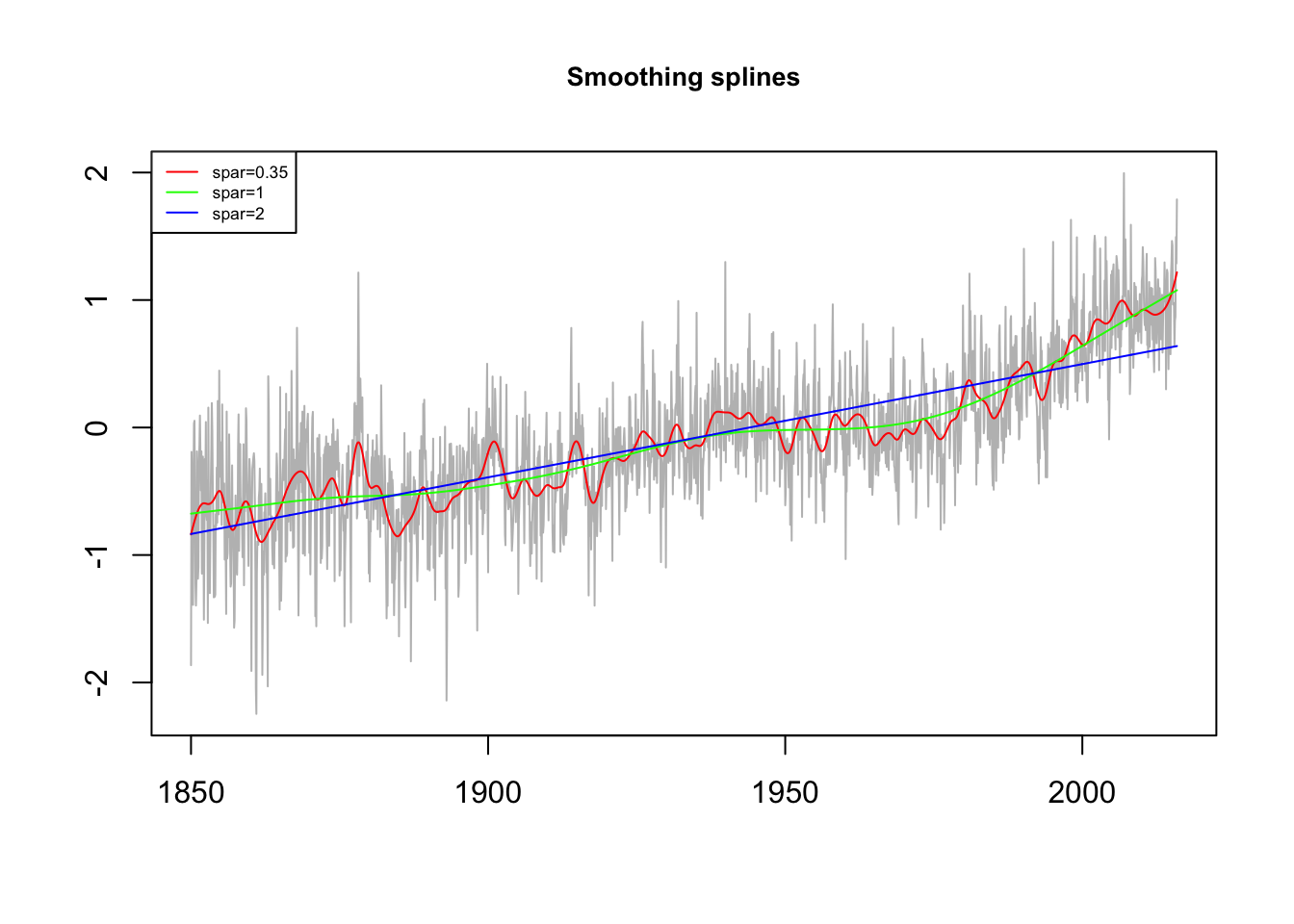Spline smoothing is an extension of polynomial regression. In spline smoothing the time $$t = 1, ..., n$$ is divided into $$k$$ intervals,$$[t_0=1,t_1], [t_i+1, t_2], ..., [t_{k-1}+1, t_k=n]$$. The values $$t_0, t_1, ...,t_k$$ are called knots. Then, in each interval a polynomial regression of the form

$f_t = \beta_0+\beta_1t+...+\beta_pt^p$ is fitted, where typically $$p=3$$, which is then called cubic spline. The regression is fitted by minimizing

$\sum_{t=1}^n (x_t-f_t)^2+\lambda\int(f^{"}_t)^2dt\text{,}$

where $$f_t$$ is a cubic spline with a knot at each $$t$$. This optimization results in a compromise between the fit and the degree of smoothness, which is controlled by $$\lambda \ge 0$$. As $$\lambda \to 0$$ (no smoothing), the smoothing spline converges to the interpolating spline, and as $$\lambda \to \infty$$ (infinite smoothing), the roughness penalty becomes paramount and the estimate converges to a linear least squares estimate (Shumway and Stoffer 2011).

In R the spline smoothing is implemented in the smooth.spline() function, which fits a cubic smoothing spline to the supplied data. In this function the smoothing parameter is called spar, and is typically (but not necessarily) in $$(0,1]$$.

library(xts)

dt <- index(temp.global)
y <- coredata(temp.global)
plot(dt, y, type = 'l',
col = 'gray', xlab = "", ylab = "",
main = 'Smoothing splines',
cex.main = 0.85)

lines(smooth.spline(dt, y, spar = 0.35),
col = 'red', type = 'l')
lines(smooth.spline(dt, y, spar = 1),
col = 'green', type = 'l')
lines(smooth.spline(dt, y, spar = 2),
col = 'blue', type = 'l')

legend('topleft',
legend = c('spar=0.35',
'spar=1',
'spar=2'),
col = c('red', 'green', 'blue'),
lty = 1,
cex = 0.55)